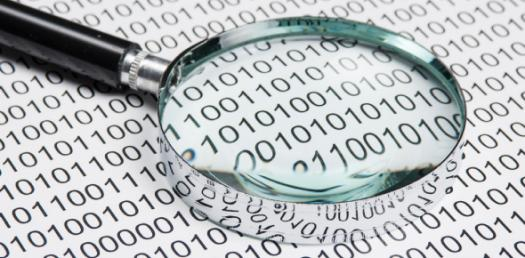# Online Mock Test 1: Number System

10 Questions | Attempts: 3571
ShareSettings• 1.
If both 112 and 33 are factors of the number a * 43 * 62 * 1311, then what is the smallest possible value of 'a'?
• A.

121

• B.

363

• C.

3267

• D.

33

• 2.
Find the greatest number of five digits, which is exactly divisible by 7, 10, 15, 21 and 28.
• A.

99840

• B.

99960

• C.

99900

• D.

99990

• 3.
1025 - 7 is divisible by
• A.

9

• B.

2

• C.

3

• D.

None of these

• 4.
When a number is divided by 36, it leaves a remainder of 19. What will be the remainder when the number is divided by 12?
• A.

10

• B.

7

• C.

192

• D.

None of these

• 5.
Find the G.C.D of 12x2y3z2, 18x3y2z4, and 24xy4z3
• A.
• B.
• C.
• D.
• 6.
Let x, y and z be distinct integers. x and y are odd and positive, and z is even and positive. Which one of the following statements cannot be true?
• A.

is even

• B.

is odd

• C.

is odd

• D.

is even

• 7.
What is the value of M and N respectively? If M39048458N is divisible by 8 & 11; Where M & N are single digit integers?
• A.

7, 8

• B.

8, 6

• C.

6, 4

• D.

5, 4

• 8.
48 students have to be seated such that each row has the same number of students as the others. If at least 3 students are to be seated per row and at least 2 rows have to be there, how many arrangements are possible?
• A.

4

• B.

10

• C.

8

• D.

7

• 9.
The sum of the first 100 numbers, 1 to 100 is divisible by
• A.

2, 4 and 8

• B.

2 and 4

• C.

2 only

• D.

None of these

• 10.
Anita had to do a multiplication. Instead of taking 35 as one of the multipliers, she took 53. As a result, the product went up by 540. What is the new product?
• A.

1050

• B.

540

• C.

1440

• D.

1590Back to top
×

Wait!
Here's an interesting quiz for you.